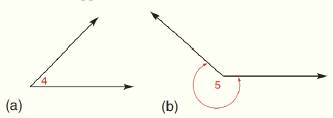Chapter 1.CR, Problem 19CR### Elementary Geometry for College St...

6th Edition
Daniel C. Alexander + 1 other
ISBN: 9781285195698

#### Solutions

Chapter
Section### Elementary Geometry for College St...

6th Edition
Daniel C. Alexander + 1 other
ISBN: 9781285195698
Textbook Problem
1 views

# On the basis of appearance, what type of angle is shown?To determine

a)

To find:

The type of angle on the basis of appearance.

Explanation

Given:

The given angle is shown as,

Figure (1)

Definition:

If the measure of an angle is less than 90° then that angle is known as acute angle.

If the measure of an angle is equal to 90° then that angle is known as right angle.

If the measure of an angle is greater than 90° and less than 180° then that angle is known as obtuse angle

To determine

To find:

The type of angle on the basis of appearance.

### Still sussing out bartleby?

Check out a sample textbook solution.

See a sample solution

#### The Solution to Your Study Problems

Bartleby provides explanations to thousands of textbook problems written by our experts, many with advanced degrees!

Get Started

#### (2, 5]

Applied Calculus for the Managerial, Life, and Social Sciences: A Brief Approach

#### Convert the expressions in Exercises 6584 to power form. xx

Finite Mathematics and Applied Calculus (MindTap Course List)

#### f(x)=12x+2

Precalculus: Mathematics for Calculus (Standalone Book)

#### True or False: div curl F = 0.

Study Guide for Stewart's Multivariable Calculus, 8th